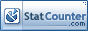ESTIMATING YOUR BREAK EVEN POINT   The break even point is the level of operations at which sales or revenue is equal to total expenses or total costs.  Thus, if profit is the excess of revenues over expenses, at the break even point there would be no profit and by the way, no loss.  Understanding the break even level of your business can be very useful, especially if you are planning to expand or decline operations.  The following equation can be used to calculate the break even point or the level of sales needed to reach a target amount of profit: S=FC + TP CM s (sales) is the dollar amount of desired sales and represents what we are trying to calculate. TP (target profit) is the desired profit and is randomly set by you.  If set at \$0, then the break even point can be calculated. FC (fixed costs) are costs that your business would incur if you had no sales.  These costs, within the relevant range, don't change relative to increases or decreases in sales volume.  An example may be the rent paid for the use of a building or salaries of office personnel.  Total costs or expenses are mixed costs and consist of the sum of fixed costs and variable costs(VC).  Variable costs  are costs and expenses that increase or decrease in total in proportion to the amount of sales increases or decreases.  For example, if you sold one toy remote helicopter for \$20 which you purchased at the wholesale price of \$10, your VC would be \$10 or 1/2 of the sales price.  The VC ratio to sales is .50.  If you sold two helicopters at \$20 each, the total VC would be .50 x \$40 or \$20.  as you can see, the total VC increased proportionately from \$10 to \$20 as sales increased from \$20 to \$40. CM (contribution margin) is the amount of each sales dollar after VC has been deducted which is available to cover fixed costs and to provide a profit, if any.  For example, if the VC ratio is .50 ( \$.50 of each sales dollar), then the contribution margin per sales dollar is \$1-.50 or \$.50.  This means that out of each sales dollar, \$.50 is available to cover FC and a profit, if any. In a new business, you will have to project your fixed costs and variable costs in order to determine your CM to be used in the aforementioned equation.  Existing businesses with a financial history may choose to use the "high-low" method to separate total mixed costs and expenses into FC and VC and to calculate the CM.  Click here for a discussion of the High-Low method and an example of calculating a break even point.© TTWS